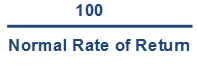# Partnership Goodwill Notes2

## Partnership - Goodwill

#### II. METHODS OF VALUATION OF GOODWILL

##### A. Simple Average Profit

Goodwill = Simple Average Profit X Number of years purchased

Where:

Simple Average profit = Simple Average of pure profit of last few years

Computation of Pure Profits

Profit or Loss of past year (before adjustments)

1. Abnormal losses (e.g., loss by fire, theft etc.)
2. Loss on sale of fixed assets
3. Overvaluation of Opening stock or Undervaluation of Closing Stock
4. Non-recurring expenses
5. Assets treated as expense less depreciation

Less:

1. Abnormal gains
2. Profit on sale of fixed assets
3. Overvaluation of Closing stock or Undervaluation of Opening Stock
4. Non-recurring incomes
5. Expenses treated as assets less depreciation
6. Partners Salary

Number of years purchased = For how many years firm will earn same profits (this is given in the question)

##### B. Weighted Average Profit

Goodwill = Weighted Average Profit X Number of years purchased

Where:

Weighted Average profit = Weighted Average of pure profit of last few years

• Weights for each year Profit is given in the question
• Pure Profit is multiplied by weight of respective year and ‘Product’ is obtained
• Weighted Average Profit = Total Product / Total Weights

Number of years purchased = For how many years firm will earn same profits (this is given in the question)

##### C. Super Profit Method

Goodwill = Super Profit X Number of years purchased

Where:

Super Profit = Average Profit – Normal profit

Average profit = Simple Average of pure profit of last few years

Normal Profit = Normal Profit is the Profit earned by similar firms in similar businesses and can be computed with the formula

Average Capital Employed X Normal Rate of Return

Average Capital Employed =

(Capital Employed at beginning of year + Capital Employed at end of year) / 2

Normal Rate of Return = Rate of return earned by the similar firms in the market. This rate is already given in the question

Number of years purchased = For how many years firm will earn same profits (this is given in the question)

Computation of Capital Employed

Liabilities Side Approach

Capital

1. Reserves

Less:

2. Goodwill

Assets Side Approach

All Assets (excluding goodwill, fictitious assets, Non-trade Investments)

Less:

1. Outside Liabilities
##### D. Capitalization of Super Profit Method

Goodwill = Super Profit XWhere:

Super Profit = Average Profit – Normal profit

Average profit = Simple Average of pure profit of last few years

Normal Profit = Profit earned by similar firms in similar businesses and can be computed with the formula:

Average Capital Employed X Normal Rate of Return

Normal Rate of Return = Rate of return earned by the similar firms in the market. This rate is already given in the question

##### E. Capitalization of Average Profits

Goodwill Employed = Average Profits X–   Net Assets or Average Capital

• As per the profit earned by firm, how much capital is required for the same (using normal rate)
• How much Capital Firm has put in the business (Capital Employed)
• If the Firm is able to earn more profits even by putting lesser capital, it is because of Firm’s Goodwill

Where:

Average Profits = Simple Average of pure profit of last few years

Normal Rate of Return = Rate of return earned by the similar firms in the market. This rate is already given in the question

error: Content is protected !!